NEET  >  Test: Imperfections in Solids

# Test: Imperfections in Solids - NEET

Test Description

## 21 Questions MCQ Test Topic-wise MCQ Tests for NEET - Test: Imperfections in Solids

Test: Imperfections in Solids for NEET 2023 is part of Topic-wise MCQ Tests for NEET preparation. The Test: Imperfections in Solids questions and answers have been prepared according to the NEET exam syllabus.The Test: Imperfections in Solids MCQs are made for NEET 2023 Exam. Find important definitions, questions, notes, meanings, examples, exercises, MCQs and online tests for Test: Imperfections in Solids below.
Solutions of Test: Imperfections in Solids questions in English are available as part of our Topic-wise MCQ Tests for NEET for NEET & Test: Imperfections in Solids solutions in Hindi for Topic-wise MCQ Tests for NEET course. Download more important topics, notes, lectures and mock test series for NEET Exam by signing up for free. Attempt Test: Imperfections in Solids | 21 questions in 30 minutes | Mock test for NEET preparation | Free important questions MCQ to study Topic-wise MCQ Tests for NEET for NEET Exam | Download free PDF with solutions
 1 Crore+ students have signed up on EduRev. Have you?
Test: Imperfections in Solids - Question 1

### Only One Option Correct Type This section contains 9 multiple choice questions. Each question has four choices (a), (b), (c) and (d), out of which ONLY ONE is correct. Q.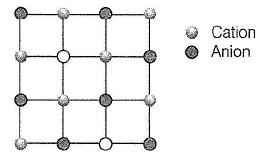Detailed Solution for Test: Imperfections in Solids - Question 1

. (a) A Schottky defect consists of a pair of holes in the crystal lattice. One positive ion and one negative ion are absent.

Note This sort of defect occurs mainly in highly ionic compounds where cation and anion are of similar size, hence the coordination number is high (8.6) as in NaCI, CsCI, KCI, KBr

Test: Imperfections in Solids - Question 2

### Structure shown in the figure represents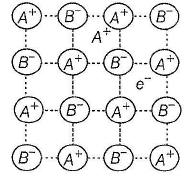Detailed Solution for Test: Imperfections in Solids - Question 2

The picture is shown as cation excess that means metal excess with the absence of anion.

Test: Imperfections in Solids - Question 3

### Which of the following defects is also known as dislocation defect?

Detailed Solution for Test: Imperfections in Solids - Question 3

(a) If there is no deficie ncy of cation or anion but cation migrates to interstitial site, it is called dislocation. It is observed in the crystals with smaller cation and larger anion, so that cation can dislocate easily. It is called Frenkel defect.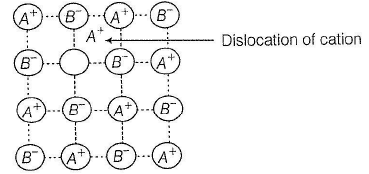Test: Imperfections in Solids - Question 4

Cations are present in the interstitial sites in

Detailed Solution for Test: Imperfections in Solids - Question 4

. (a) Frenkel defect is observed in the crystals in which cation is much smaller than the anion so that cation can migrate to interstitial position.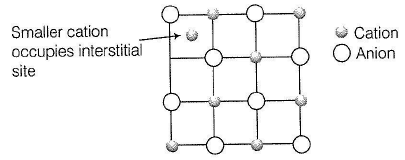Test: Imperfections in Solids - Question 5

Select the correct statement about non-stoichiometric compounds

Detailed Solution for Test: Imperfections in Solids - Question 5

(a)  Non-stoichiometric compounds are also called Berthollide compounds thus, correct.

(b) Since, composition of the constituent elements is not fixed, thus law of constant composition is not followed as is Fe 0,98 O thus, correct.

(c) If cation (say A+) is missing then, charge is balanced by the cation A2+. If anion (say B-) is missing, then charge is balanced by inserting electron (e-)thus, correct.

Test: Imperfections in Solids - Question 6

In a face-centred cubic lattice, atom A occupies the corners of the cube and atom B occupies the face-centred positions. If one atom of B is missing from one of the face-centred points, the formula of the compound is

Detailed Solution for Test: Imperfections in Solids - Question 6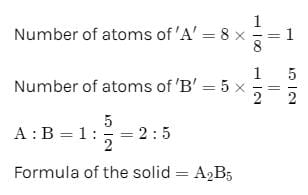Test: Imperfections in Solids - Question 7

An element crystallises in fcc lattice having edge length 40 0 pm. Maximum radius of the atom which can be placed in the interstitial site without distorting the structure is

Detailed Solution for Test: Imperfections in Solids - Question 7

(a) For fcc structure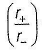= 0.414 for octahedral void

= 0.225 for tetrahedral void

Thus, maximum packing can be for octahedral void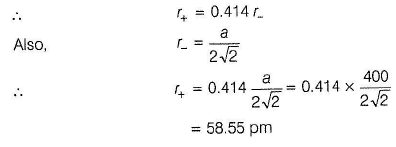Test: Imperfections in Solids - Question 8

Experimentally it was found that a metal oxide has formula M0.98O. Metal M, is present as M2+ and M3+ in its oxide. The fraction of the metal which exists as M3+ would be:

Detailed Solution for Test: Imperfections in Solids - Question 8

Metal oxide = M0.98O
If ‘x’ ions of M are in +3 state, then
3x + (0.98 – x) × 2 = 2
x = 0.04
So the percentage of metal in +3 state would be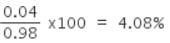Test: Imperfections in Solids - Question 9

If NaCI is doped with 10-3 mole % of SrCI2 then , number of cation ic vacancies is

Detailed Solution for Test: Imperfections in Solids - Question 9

(c)

Due to the addition of SrCI2, each Sr2+ ion replaces two Na+ ions, but occupies one Na+ lattice point. Thus, this exchange of Na+ ion by Sr2+ ion makes one cationic vacancy.

SrCI2 doped = 10-3 mol per 100 mol = 10-5 mol per 1 mol

∴ Cation vacancies = 10-5 mol per 1 mol

= 10-5 x N0 mol-1

= 10-5 x 6.02 x 1023

Total = 6.02 x 1018 cationic vacancies mol-1

*Multiple options can be correct
Test: Imperfections in Solids - Question 10

One or More than One Options Correct Type
This section contains 7 multiple type questions. Each question has 4 choices (a), (b), (c) and (d), out of which ONE or MORE THAN ONE are correct.

Q.

AgBr(s) crystals can exhibit one or more types of defect which are

Detailed Solution for Test: Imperfections in Solids - Question 10

(a, b) AgBr is a covalent c om pound with smaller A g+ (= 115 pm) and larger Br-(≈196 pm) thus, Ag+ ion can be migrated to interstitial site showing Frenkel defect. In AgBr, there is also Schottky defect per1016 ions.

Test: Imperfections in Solids - Question 11

Substitution of foreign atom in the site of parent atom in crystal is a?

Detailed Solution for Test: Imperfections in Solids - Question 11

In Substitution impurity, foreign atom takes the position of parent atom. Toatal number of atoms remains same after the defect.

Test: Imperfections in Solids - Question 12

As grain size of metal increases, Strength of metal:

Detailed Solution for Test: Imperfections in Solids - Question 12

Strength of metal is inversely proportional to size of grain. Coarse are softer in nature while material having fine grain structure are hard in nature.

*Multiple options can be correct
Test: Imperfections in Solids - Question 13

Select the correct statements about the given structure.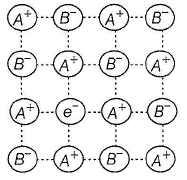Detailed Solution for Test: Imperfections in Solids - Question 13

. (a,b,c,d)

(a) Since, number of cations > number of anions, hence it I represents metal-excess defect. It is similar to Schottky defect i in which equal number of cations and anions are missing | thus, correct.

(b) Charge is balanced by electrons, these are called F-centres (farbe, colour producing). Compounds with F-centres are ! coloured. Greater the number of F-centres, greater the ! intensity thus, correct.

(c) Due to the unpaired electrons these are paramagnetic thus, correct. j:

(d) When NaCI, KCl, etc., are strongly heated in flame, they become deficient in anions and represented as AS, _ s where, § is small fraction.
Non-stoichiometric form of NaCl is yellow and that is KClis blue-lilac thus, correct

*Multiple options can be correct
Test: Imperfections in Solids - Question 14

Select the correct statement(s) about following structure.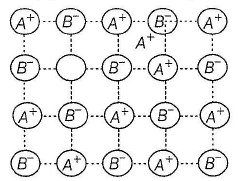Detailed Solution for Test: Imperfections in Solids - Question 14

(a) correct
(b) As cation (A' ) occupies interstitial position, thus cation is smaller than anion, thus correct.
(c) Due to the smaller cation and larger anion, coordination number Is usually low (4, 6), thus correct.
(d) By Fajans' rule, smaller cations polarise larger anions thus, compounds are covalent, thus correct.

*Multiple options can be correct
Test: Imperfections in Solids - Question 15

Which of the following statement(s) is/are correct?

Detailed Solution for Test: Imperfections in Solids - Question 15

(a, b)

(a) When cation, anion or both are missing, molar mass is y ass decreased. Since, density = Mass/ Volume. hence density Volume decreases, thus correct.

(b) When there is insertion of cation (A + ) in the interstitial site, it causes metal-excess defect. Thus, molar mass increases, hence density increases, thus correct.

(c) Presence of impurity increases molar mass (as doping of metal with P or Si), hence density changes, thus incorrect.

(d) In Frenkel defect, cation is dislocated without affecting molar mass thus, no change in density , thus correct

Test: Imperfections in Solids - Question 16

Comprehension Type
This section contains a passage describing theory, experiments, data, etc. Two questions related to the paragraph have been given. Each question has only one correct answer out of the given 4 options (a), (b), (c) and (d).

Passage I

The crystal AB (rock salt structure) has m ole cular w eight 6.02 y u, where y is an arbitrary unit in u. Also, given minimum distance between cation and anion = y 1/3 nm and observed density = 20 kg m-3.

Q.

Theoretical density of AB is

Detailed Solution for Test: Imperfections in Solids - Question 16

(b)

AB is rock salt like structure, thus number of AB molecule per
unit cell = 4, a = 2y1/3 nm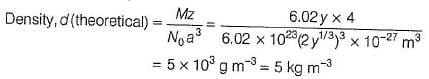Obseved value of d(20 kgm-3) >>5kgm-3

Thus, there is excess of metal ions which incerease mass hence the density

Test: Imperfections in Solids - Question 17

Passage

The crystal AB (rock salt structure) has m ole cular w eight 6.02 y u, where y is an arbitrary unit in u. Also, given minimum distance between cation and anion = y 1/3 nm and observed density = 20 kg m-3.

Q.

Defect present in the crystal is of the type

Detailed Solution for Test: Imperfections in Solids - Question 17

AB is rock salt like structure, thus number of AB molecules per unit cell  =4, a = 2y1/3 nm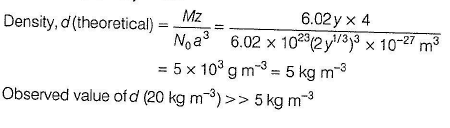Thus, there is excess of metal ions which increases mass hence the density.

Test: Imperfections in Solids - Question 18

Passage II

Titanium (II) oxide has a rock salt structure. X-ray diffraction data shows that the length of one edge of the cubic unit cell for TiO with 1:1 ratio of Ti to O is 418 pm and the density is 4.92 g cm-3. ( Atomic mass of Ti = 48).

Q.

Defect present in the crystal is of the type

Detailed Solution for Test: Imperfections in Solids - Question 18

Rock salt (NaCl) is fcc type structure, z = 4

M = 64 g mol-1, a = 418 pm

theoretical density (d) =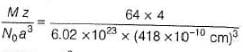= 5.82 g cm-3

Experimental density = 4.92 g cm-3

thus, experimental density << theoretical density

thus, there is vacancy in the crystal

d= Mass / Volume

Due to decrease in density, molar mass should decrease. this indicates Schottky defect.

Let Schottky defect be x%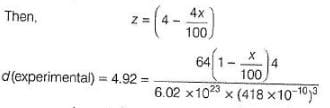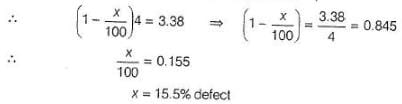Test: Imperfections in Solids - Question 19

Passage II

Titanium (II) oxide has a rock salt structure. X-ray diffraction data shows that the length of one edge of the cubic unit cell for TiO with 1:1 ratio of Ti to O is 418 pm and the density is 4.92 g cm-3. ( Atomic mass of Ti = 48).

Q.

% of vacancies (defects) in the structure is

Detailed Solution for Test: Imperfections in Solids - Question 19

Rock salt (NaCl) is fcc type structure, z = 4

M = 64 g mol-1, a = 418 pm

theoretical density (d) == 5.82 g cm-3

Experimental density = 4.92 g cm-3

thus, experimental density << theoretical density

thus, there is vacancy in the crystal

d= Mass / Volume

Due to decrease in density, molar mass should decrease. this indicates Schottky defect.

Let Schottky defect be x%Test: Imperfections in Solids - Question 20

Matching List Type
Choices for the correct combination of elements from Column I and Column II are given as options (a), (b), (c) and (d), out of which one is correct.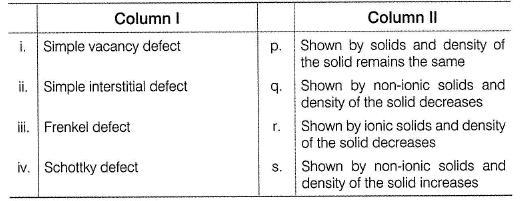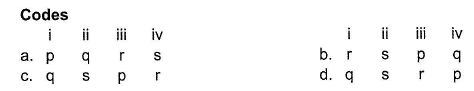Detailed Solution for Test: Imperfections in Solids - Question 20

(i) When cation or anion is absent, molar mass decreases hence, density decreases.
Thus, (i) - » (q)

(ii) When cation is packed in the interstitial site, molar mass increases. Hence, density increases.
Thus, (ii) - » (s)

(iii) When smaller cation migrates into the interstitial site, Frenkel defect is observed. There is no change in molar mass and thus, no change in density.
Thus, (iii) -> (p)

(iv) As in NaCI, when cation and anion are missing (Schottky defect), molar mass and thus, density decreases.
Thus, (iv) - 0

*Answer can only contain numeric values
Test: Imperfections in Solids - Question 21

One Integer Value Correct Type
This section contains 1 question, when worked out will result in an integer value from 0 to 9 (both inclusive)

Q.

Calcium crystallises in a face-centred cubic unit cell with a = 0.556 nm and density 1.4848 g/cm3. Percentage of Schottky defects in this crystal is ... .

Detailed Solution for Test: Imperfections in Solids - Question 21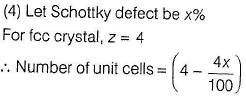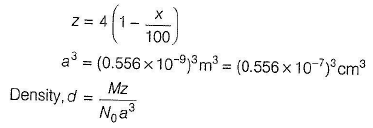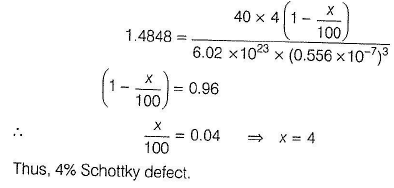## Topic-wise MCQ Tests for NEET

9 docs|1470 tests
Information about Test: Imperfections in Solids Page
In this test you can find the Exam questions for Test: Imperfections in Solids solved & explained in the simplest way possible. Besides giving Questions and answers for Test: Imperfections in Solids, EduRev gives you an ample number of Online tests for practice

## Topic-wise MCQ Tests for NEET

9 docs|1470 tests

### How to Prepare for NEET

Read our guide to prepare for NEET which is created by Toppers & the best Teachers